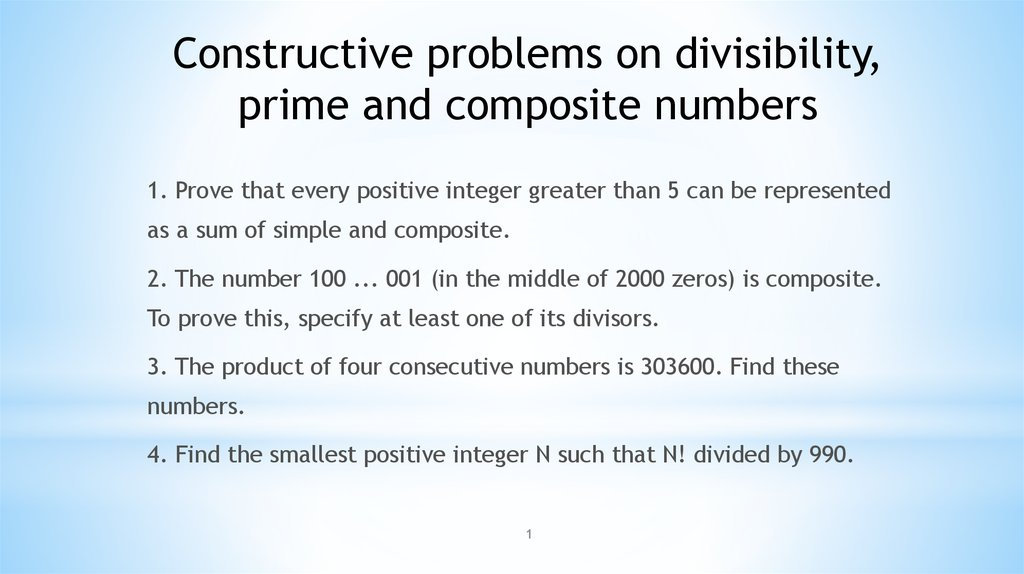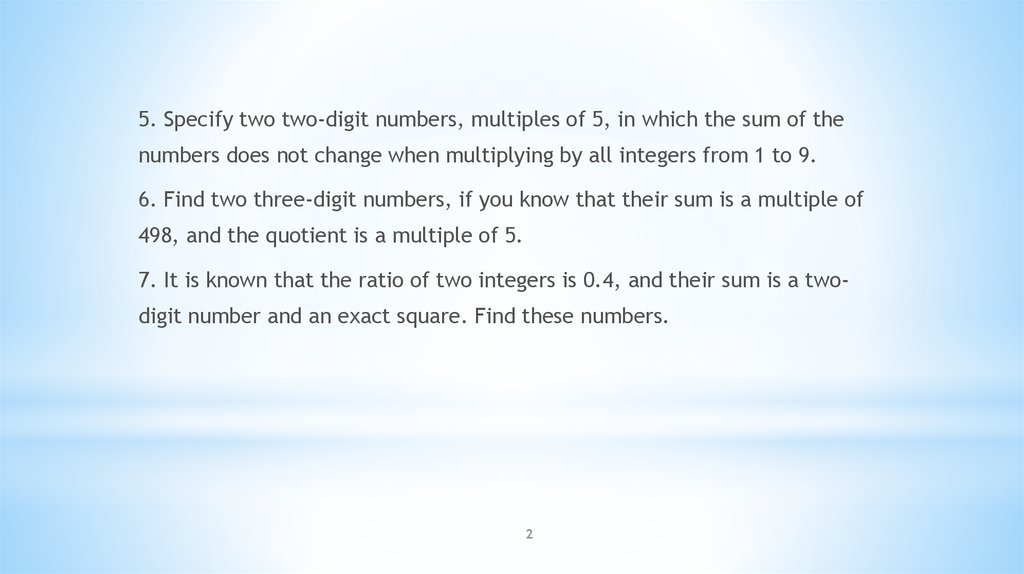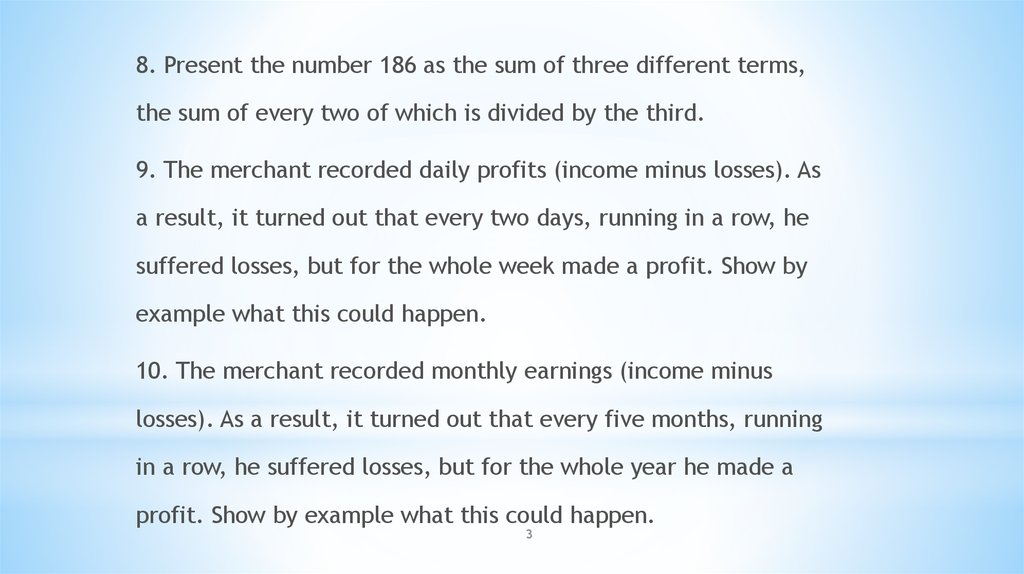Сonstructive problems on divisibility, prime and composite numbers

1.

Сonstructive problems on divisibility,
prime and composite numbers
1. Prove that every positive integer greater than 5 can be represented
as a sum of simple and composite.
2. The number 100 ... 001 (in the middle of 2000 zeros) is composite.
To prove this, specify at least one of its divisors.
3. The product of four consecutive numbers is 303600. Find these
numbers.
4. Find the smallest positive integer N such that N! divided by 990.
1

2.

5. Specify two two-digit numbers, multiples of 5, in which the sum of the
numbers does not change when multiplying by all integers from 1 to 9.
6. Find two three-digit numbers, if you know that their sum is a multiple of
498, and the quotient is a multiple of 5.
7. It is known that the ratio of two integers is 0.4, and their sum is a twodigit number and an exact square. Find these numbers.
2

3.

8. Present the number 186 as the sum of three different terms,
the sum of every two of which is divided by the third.
9. The merchant recorded daily profits (income minus losses). As
a result, it turned out that every two days, running in a row, he
suffered losses, but for the whole week made a profit. Show by
example what this could happen.
10. The merchant recorded monthly earnings (income minus
losses). As a result, it turned out that every five months, running
in a row, he suffered losses, but for the whole year he made a
profit. Show by example what this could happen.
3

4.

11. Is there a set of four weights with a total weight of 40 kg, such that any
whole number of kilograms from 1 to 40 kg can be weighed on the pan
weights?
12. Find 10 natural numbers, the sum of which is equal to the product.
13. The product of two two-digit numbers consists of only fours. Find these
numbers.
14. Indicate two consecutive positive integers, the sum of the digits of each
of which is a multiple of 7.
15. Find a four-digit number that, when you rearrange the numbers in the
reverse order, increases 4 times.
4

5.

16. Turn a 3x3 square by 45 degrees and place the numbers from 1 to
9 (each 1 time) in it so that horizontally it turns out 5 exact squares.
17. In a 3x3 square, arrange the numbers from 1 to 9 (each 1 time), so
that horizontally you get 3 exact squares.
18. Give an example of such a number that if you add 10 to it, you get
an exact cube, and if you subtract 10, you get an exact square.
19. Is there a set of positive numbers, the sum of all numbers is at
least 10, and the sum of squares is not more than 0.01?
20. In a four digit number, the first digit is equal to the second, and
the third one is equal to the fourth. In addition, the number is an
exact square. Find this number.
5

6

7.

Strassen algorithm is an algorithm
for matrix multiplication
7

8

9

10